# Tips from an Online Math Tutor: Stop Using F.O.I.L Now – Before It’s Too Late!

Math students across the country have heard the mnemonic acronym FOIL from their school teachers. It’s a tactic for calculating the product of two binomials that stands for First, Outside, Inside, Last. Here is an example of FOIL in action for multiplying the terms (x + 2) and (x + 3):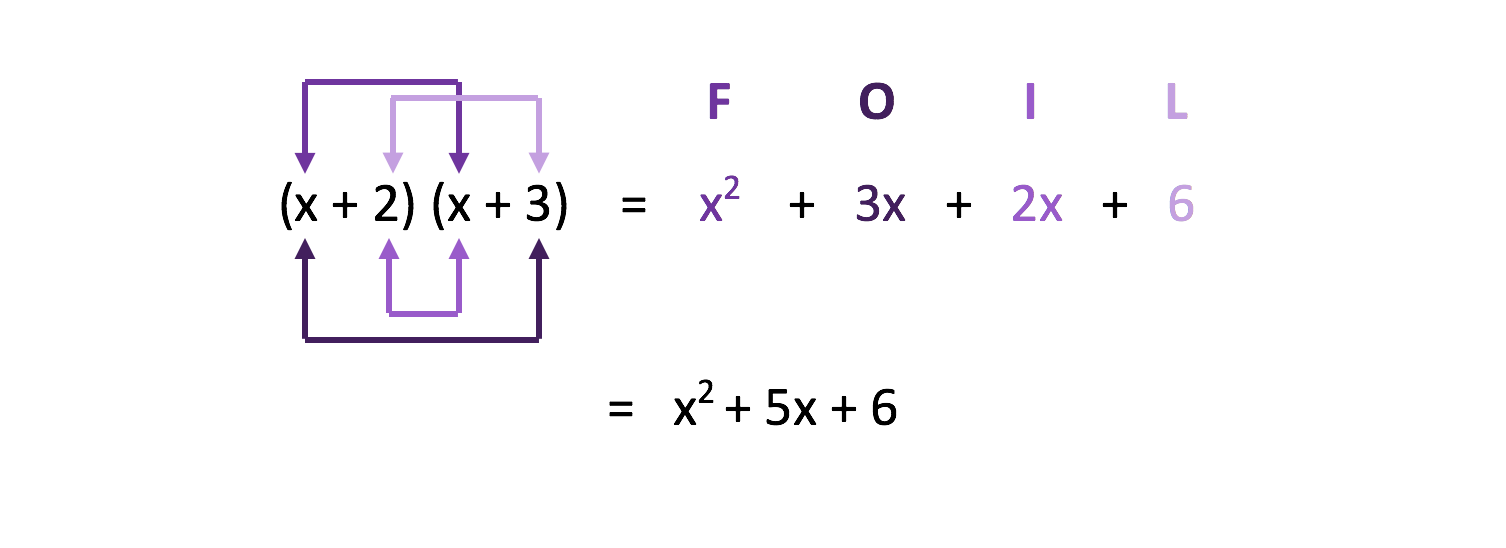Here we can see that the “first” terms of each binomial (the x and the second x) are multiplied together in orange to get x2. Then the “outside” terms (the first x and the 3) are multiplied in yellow to get 3x. Then the “inside” terms (the 2 and the second x) are multiplied in blue to get 2x. And finally, the “last” terms of each binomial (the 2 and the 3) are multiplied in green to get 6. We add those four terms together to get our final answer of x2 + 5x + 6.

So far this looks like a good mnemonic. We got the answer we were looking for. So, what’s the problem?

The issue is that FOIL only works for two binomials. As you continue in math, you will be multiplying many other terms together. It could be a binomial with a trinomial, it could be three binomials together or any different combination of polynomials. Consider these examples: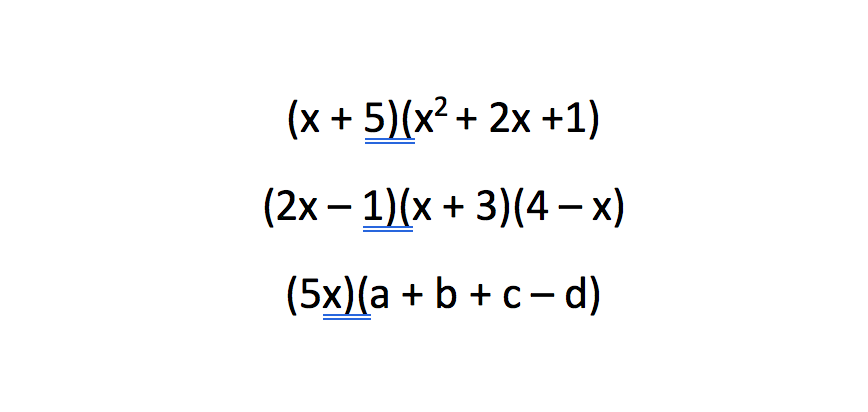FOIL will not work for any of these cases, and memorizing it and “FOIL-ing” everything will prevent you from learning and mastering the math skill actually in play.  And guess what?  If you’ve made it to FOIL, you’ve already learned the math you need: the distributive property.

Here is an example you probably already know using the distributive property: 3(x-2)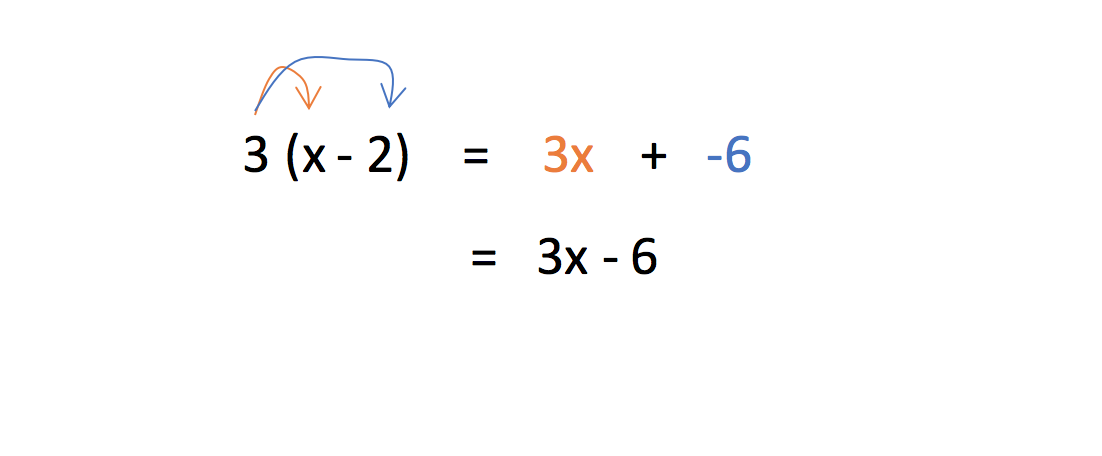Notice how we took the 3 that was being multiplied to the term in the parentheses and we distributed it (multiplied it) to each term inside of the parentheses.
We can use this same tactic instead of FOIL-ing! The only difference is we might have more than just a “3” that we need to distribute. Let’s re-do the first example that we used FOIL on. There are two terms inside of the first parentheses, so we just need to distribute both of them.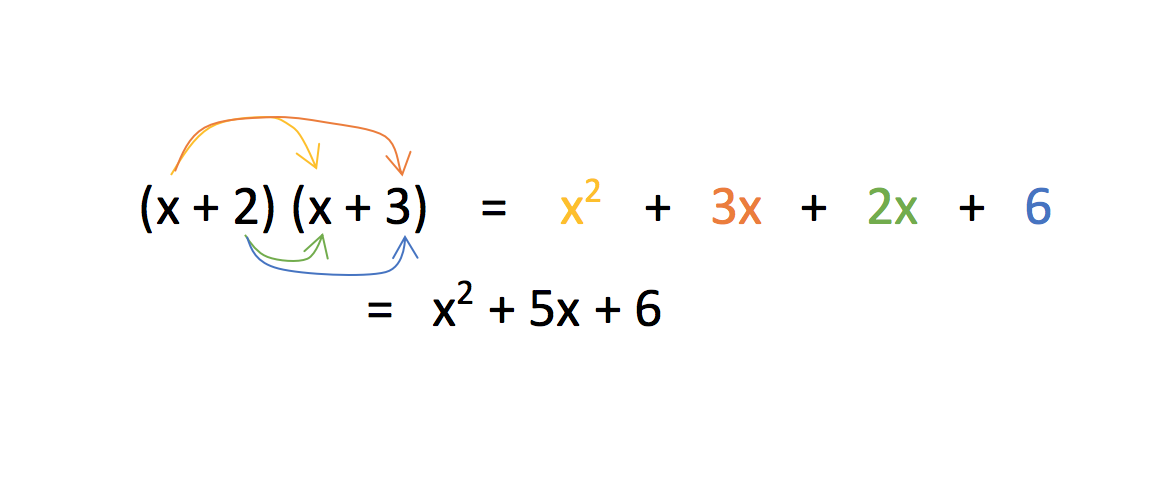We get the same answer as to when we used FOIL, but it doesn’t matter what order we do it in.  We just took the x and distributed it to the (x+3) (on the top) and we took the +2 and distributed it to the (x+3) (on the bottom) as well.  No mnemonic, no memorization – just the distributive property that we already know.

Now let’s use this distributive property skill on the examples above that were impossible to FOIL.  FOIL fails us here, but watch how simple, though sometimes tedious, it is to just distribute.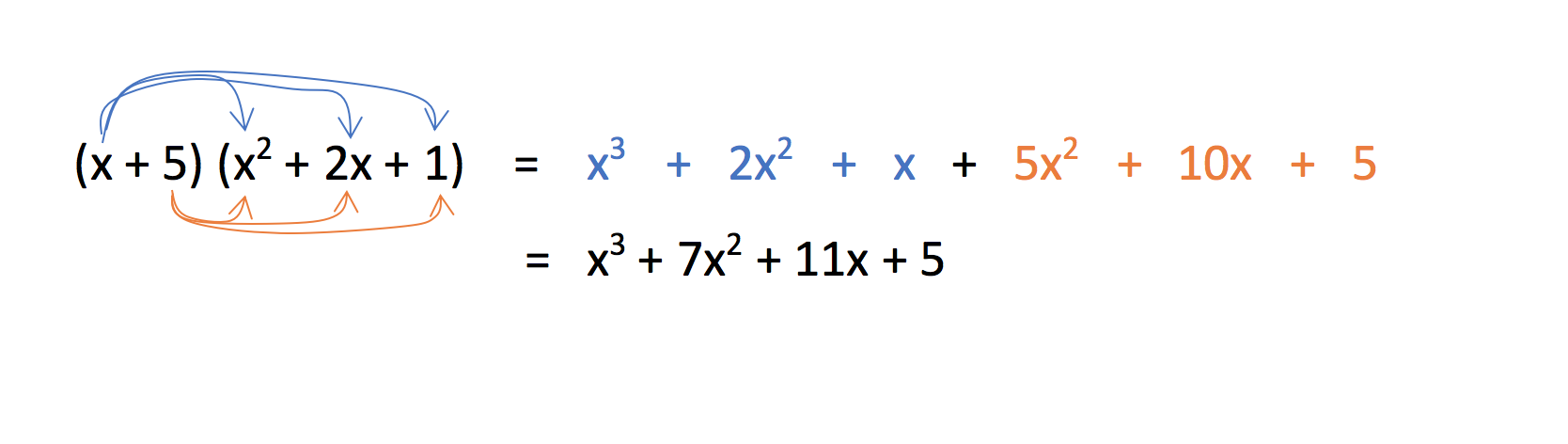We multiplied the x to all three terms inside the second parentheses (top, blue) and then multiplied the 5 to all three terms in the second parentheses (bottom, orange). First, Outside, Inside, Last wouldn’t have worked here.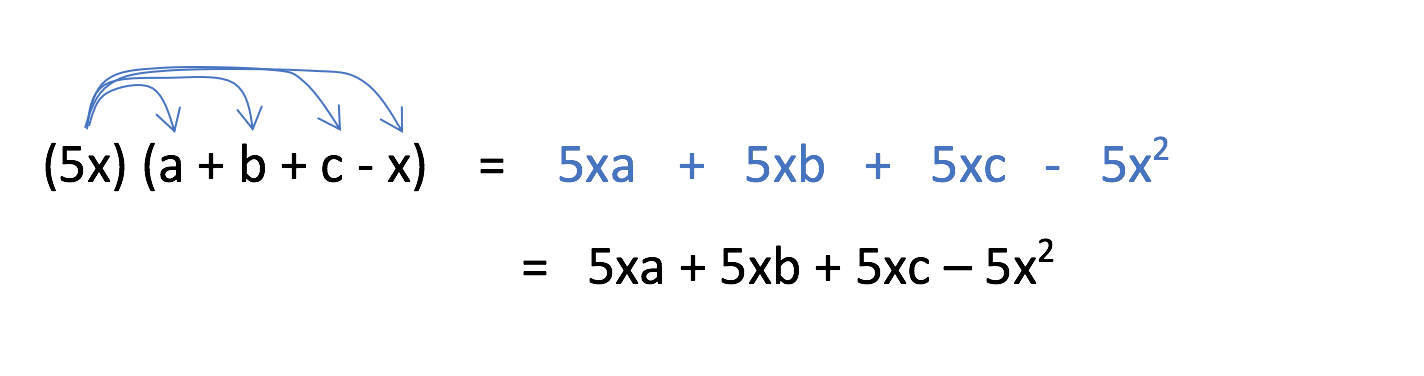5x is just one term, so we simply distribute it to all four terms inside.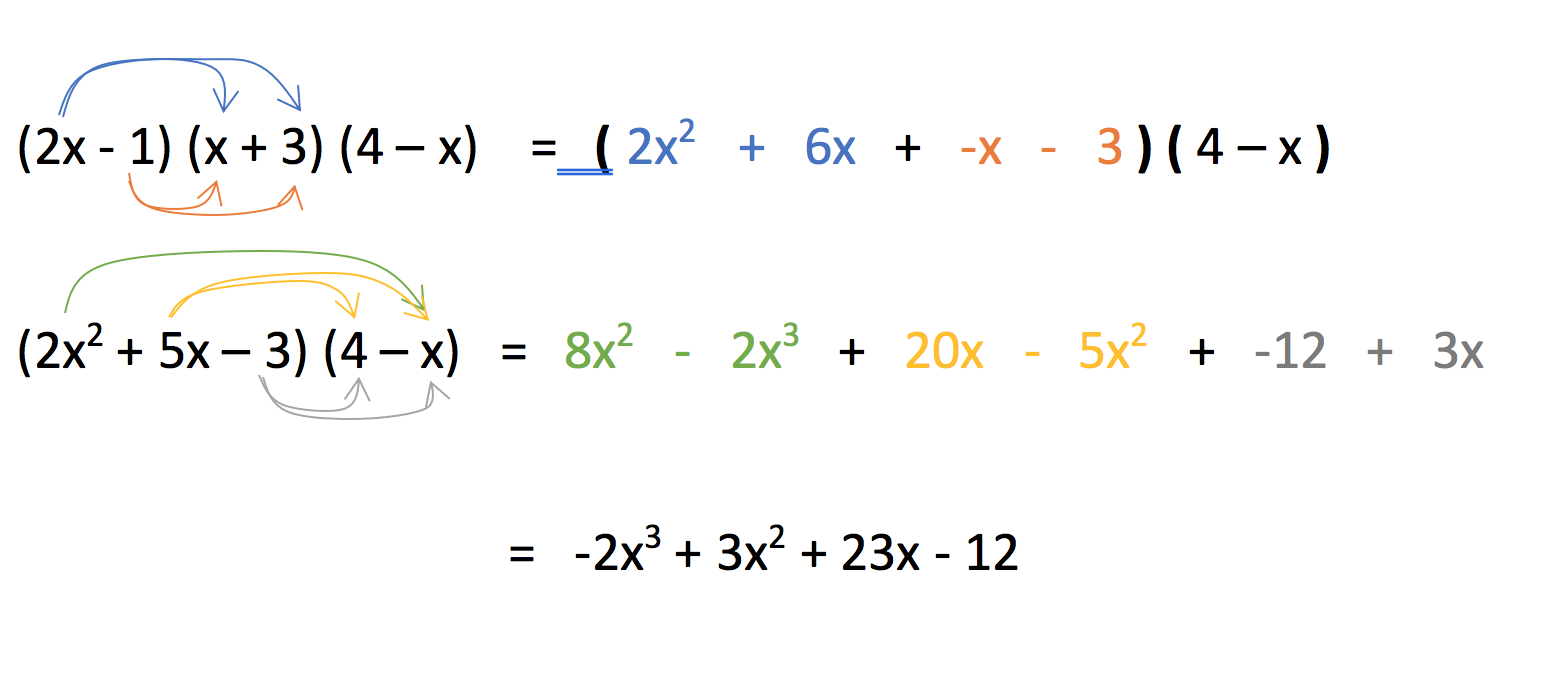Here, we needed to distribute twice. FOIL may have worked for the first time, but wouldn’t for the second time. First, we distributed both terms inside (2x-1) to both terms of the (x+3) (blue and orange). We saved the (4-x) to do second. Out result from the first distribution was (2x2+5x-3). We kept it in parentheses and distributed all three terms to our remaining two terms inside the (4-x) (green for the 2x2 multiplication, yellow for the 5x multiplication, and grey for the -3 multiplication).

Now, we could have done the previous example in several ways. We could have multiplied the second and third parentheses first instead of the first and second. We also could have distributed the (4-x) in the last step instead of the (2x2+5x-3). There are many ways you can do the order of distribution, as long as you multiply and simplify each term properly, you should always get the same answer.

FOIL will get the job done for multiplying binomials. But why memorize some arbitrary acronym when instead we can use a skill that we already know, that we can continue to master, and that works in every situation instead of just one. Don’t just memorize in math class, make sure you understand what’s going on so you aren’t lost in the future.

Michael C. is currently a private math, science, and standardized test tutor with TutorNerds in Irvine and Anaheim.